# Vector Addition as Net Effect

Suppose that a particle undergoes two displacements, the first one represented as $$\overrightarrow a$$, which is 4 meters towards the east, and the second one represented as $$\overrightarrow b$$, which is 4 meters towards the north: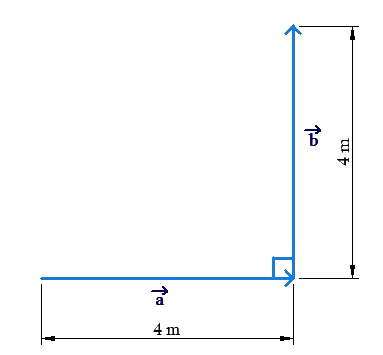To calculate the total distance travelled by the particle, we simply add the magnitudes of the two displacements: $$\left| {\overrightarrow a } \right| + \left| {\overrightarrow b } \right| = 8$$ meters.

However, what is the net effect of the two displacements? The net effect is that relative to its initial position, the particle is at a distance of $$4\sqrt 2$$ meters in the north-east direction: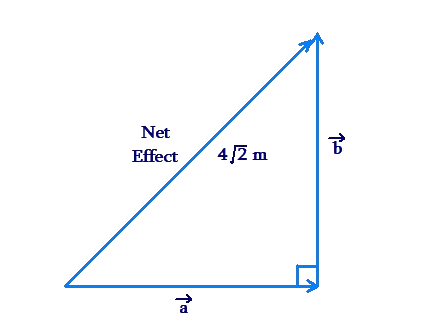Thus, the net displacement of the particle is $$4\sqrt 2$$ meters, north-east. Observe that:

• the net distance travelled is a scalar quantity, and equal to $$\left| {\overrightarrow a } \right| + \left| {\overrightarrow b } \right| = 8$$ meters.
• the net displacement is a vector quantity. Since it is the result of vectors $$\overrightarrow a$$ and $$\overrightarrow b$$ combined, we can represent the net displacement as $$\overrightarrow a + \overrightarrow b$$. The value of this net displacement is $$4\sqrt 2$$ meters, north-east. Note that this + sign in $$\overrightarrow a + \overrightarrow b$$ does not represent normal addition, in the way we think about addition. It represents vector addition – it is calculating the net effect of two vectors.

To put it differently:

• . $$\left| {\overrightarrow a } \right| + \left| {\overrightarrow b } \right|$$. is your normal, scalar, addition. In this case, $$\left| {\overrightarrow a } \right| + \left| {\overrightarrow b } \right|\, = \,8$$ meters.
• $$\overrightarrow a + \overrightarrow b$$ is vector addition, and is equal to the net effect of vectors $$\overrightarrow a$$ and $$\overrightarrow b$$. In this case, $$\overrightarrow a + \overrightarrow b$$  $$= \,4\sqrt 2$$ meters, north-east.

Exampl 1: Consider three vectors:

$$\overrightarrow a$$: 2 units, east

$$\overrightarrow b$$: 2 units, north

$$\overrightarrow c$$: 2 units, west

Find

(i) $$\left| {\overrightarrow a } \right| + \left| {\overrightarrow b } \right|$$         (ii) $$\left| {\overrightarrow a } \right| + \left| {\overrightarrow c } \right|$$      (iii) $$\left| {\overrightarrow b } \right| + \left| {\overrightarrow c } \right|$$

(iv) $$\left| {\overrightarrow a } \right| + \left| {\overrightarrow b } \right| + \left| {\overrightarrow c } \right|$$       (v) $$\overrightarrow a + \overrightarrow b$$

(vi) $$\overrightarrow a + \overrightarrow c$$       (vii) $$\overrightarrow b + \overrightarrow c$$        (viii) $$\overrightarrow a + \overrightarrow b + \overrightarrow c$$

Solution: We note that $$\left| {\overrightarrow a } \right| = \left| {\overrightarrow b } \right| = \left| {\overrightarrow c } \right| = 2$$ units, and thus the answer for each of (i), (ii) and (iii) is 4 units, while the answer for (iv) is 6 units.

(v) Now, let us determine $$\overrightarrow a + \overrightarrow b$$. Observe the following figure: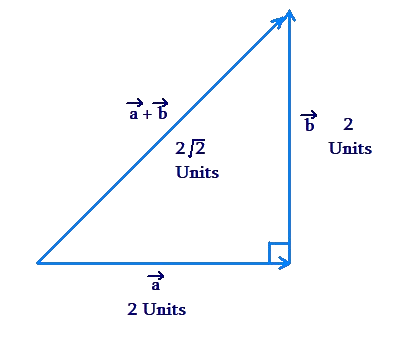Clearly, $$\overrightarrow a + \overrightarrow b = 2\sqrt 2$$ units, north-east.

(vi) The following figure shows $$\overrightarrow a$$ and $$\overrightarrow c$$: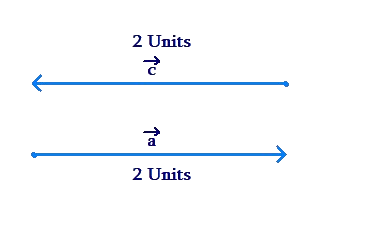What will be the net effect of these two vectors? Intuitively, it should be obvious to you that they will cancel each other out, and thus the result will be a vector of magnitude 0 units. However, no particular direction will exist for this vector. We can write this as follows: $$\overrightarrow a + \overrightarrow c = 0$$ units, unspecified direction, or $$\overrightarrow a + \overrightarrow c = \vec 0$$

(vii) The following figure shows $$\overrightarrow b$$ and $$\overrightarrow c$$, and the net effect of $$\overrightarrow b$$ and $$\overrightarrow c$$: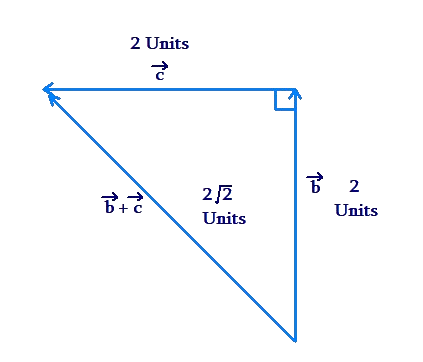Clearly, $$\overrightarrow b + \overrightarrow c = 2\sqrt 2$$ units, north-west.

(viii) The following figure shows $$\overrightarrow a$$, $$\overrightarrow b$$ and $$\overrightarrow c$$: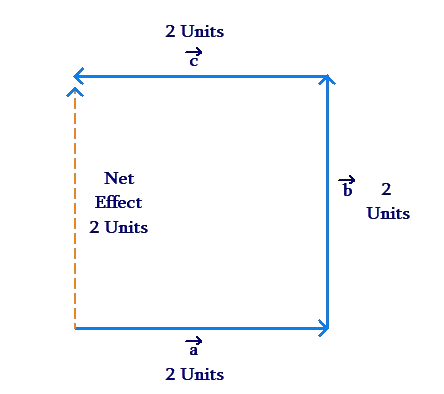What is the net effect of all the three vectors taken together? Since $$\overrightarrow a$$ and $$\overrightarrow c$$ cancel the effect of each other out, we have:

$$\overrightarrow a + \overrightarrow b + \overrightarrow c = \overrightarrow b = \,2$$ units, north